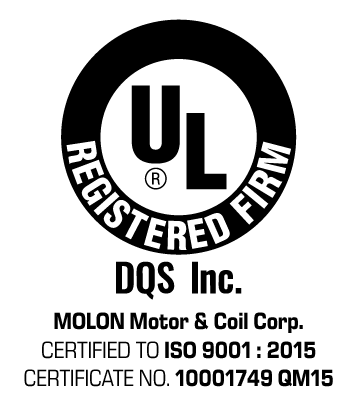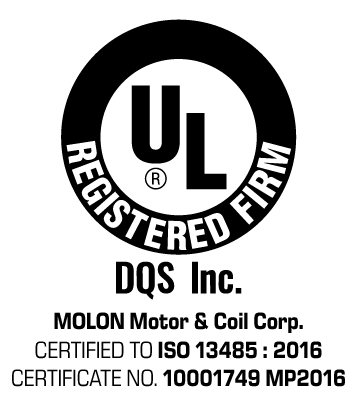PHONE:   (847) 253-6000
TOLL FREE:
(800) 52-MOTOR### Torque:

Multiply number of:

Newton-meters
(N-m)
Gram-centimeters
(g-cm)
inch-pounds
(in-lb)
inch-ounces
(in-oz)
To get:
Newton-meters (N-m) 1 0.000098 0.11299 0.00706
Gram-centimeters (g-cm) 10197 1 1152.16 72.01
inch-pounds (in-lb) 2265.76 0.22224 1 16
inch-ounces (in-oz) 141.61 0.01389 0.0625 1

1 inch = 25.4mm

1 foot = 0.305m

### Power

1 Horsepower = 746 Watts

Horsepower (Hp) = Torque (oz-in.) x Speed (rpm) = Torque (lb-in) x Speed (rpm)
10080400 63025

### Temperature:

F = (C X 9/5) + 32

C = (F - 32) X 5/9FORMULAS & CONVERSIONS